Courses

# RD Sharma Solutions (Part - 2) - Ex-20.1, Mensuration - I, Class 7, Math Class 7 Notes | EduRev

## Class 7: RD Sharma Solutions (Part - 2) - Ex-20.1, Mensuration - I, Class 7, Math Class 7 Notes | EduRev

The document RD Sharma Solutions (Part - 2) - Ex-20.1, Mensuration - I, Class 7, Math Class 7 Notes | EduRev is a part of the Class 7 Course RD Sharma Solutions for Class 7 Mathematics.
All you need of Class 7 at this link: Class 7

#### QUESTION 10:

A table top is 9 dm 5 cm long 6 dm 5 cm broad. What will be the cost to polish it at the rate of 20 paise per square centimetre?

We have,
Length of the table top = 9 dm 5 cm = (9 x 10 + 5) cm = 95 cm    [ Since 1 dm = 10 cm]
Breadth of the table top = 6 dm 5 cm = (6 x 10 + 5) cm = 65 cm
∴ Area of the table top = Length x Breadth = (95 cm x 65 cm) = 6175 cm2
Rate of polishing per square centimetre = 20 paise = Rs. 0.20
Total cost = Rs. (6175 x 0.20) = Rs. 1235

#### Question 11:

A room is 9.68 m long and 6.2 m wide. Its floor is to be covered with rectangular tiles of size 22 cm by 10 cm. Find the total cost of the tiles at the rate of Rs 2.50 per tile.

We have,
Length of the floor of the room = 9.68 m
Breadth of the floor of the room = 6.2 m
Area of the floor = 9.68 m x 6.2 m = 60.016 m2
Length of the tile = 22 cm
Breadth of the tile = 10 cm
Area of one tile = 22 cm x 10 cm = 220 cm2 = 0.022 m2    [Since 1 m2 = 10000 cm2]
Thus,
Number of tiles =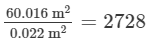Cost of one tile = Rs. 2.50
Total cost = Number of tiles x Cost of one tile
= Rs. (2728 x 2.50) = Rs. 6820

#### Question 12:

One side of a square field is 179 m. Find the cost of raising a lown on the field at the rate of Rs 1.50 per square metre.

We have,
Side of the square field = 179 m
Area of the field = (Side)2 = (179 m)2 = 32041 m2
Rate of raising a lawn on the field per square metre = Rs. 1.50
Thus,
Total cost of raising a lawn on the field = Rs.(32041 x 1.50) = Rs. 48061.50

#### Question 13:

A rectangular field is measured 290 m by 210 m. How long will it take for a girl to go two times round the field, if she walks at the rate of 1.5 m/sec?

We have,
Length of the rectangular field = 290 m
Breadth of the rectangular field = 210 m
Perimeter of the rectangular field = 2(Length + Breadth)
= 2(290 + 210) = 1000 m
Distance covered by the girl = 2 x Perimeter of the rectangular field
= 2 x 1000 = 2000 m

The girl walks at the rate of 1.5 m/sec.
or,
Rate = 1.5 x 60 m/min = 90 m/min
Thus,
Required time to cover a distance of 2000 m =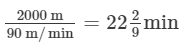Hence, the girl will take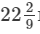min to go two times around the field.

#### Question 14:

A corridor of a school is 8 m long and 6 m wide. It is to be covered with convas sheets. If the available canvas sheets have the size 2 m × 1 m, find the cost of canvas sheets required to cover the corridor at the rate of Rs 8 per sheet.

We have,
Length of the corridor = 8 m
Breadth of the corridor = 6 m
Area of the corridor of a school = Length x Breadth = (8 m x 6 m) = 48 m2
Length of the canvas sheet = 2 m
Breadth of the canvas sheet = 1 m
Area of one canvas sheet = Length x Breadth = (2 m x 1 m) = 2 m2
Thus,
Number of canvas sheets =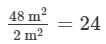Cost of one canvas sheet = Rs. 8
∴ Total cost of the canvas sheets = Rs. (24 x 8) = Rs. 192

#### Question 15:

The length and breadth of a playground are 62 m 60 cm and 25 m 40 cm respectively. Find the cost of turfing it at Rs 2.50 per square metre. How long will a man take to go three times round the field, if he walks at the rate of 2 metres per second.

We have,
Length of a playground = 62 m 60 cm = 62.6 m    [ Since 10 cm = 0.1 m]
Breadth of a playground = 25 m 40 cm = 25.4 m
Area of a playground = Length x Breadth= 62.6 m x 25.4 m = 1590.04 m2
Rate of turfing = Rs. 2.50/m2
∴ Total cost of turfing = Rs. (1590.04 x 2.50) = Rs. 3975.10

Again,
Perimeter of a rectangular field = 2(Length + Breadth)
= 2(62.6 + 25.4) = 176 m
Distance covered by the man in 3 rounds of a field = 3 x Perimeter of a rectangular field
= 3 x 176 m = 528 m
The man walks at the rate of 2 m/sec.
or,
Rate = 2 x 60 m/min = 120 m/min

Thus,
Required time to cover a distance of 528 m =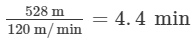=  4 minutes 24 seconds      [ since 0.1 minutes = 6 seconds]

The document RD Sharma Solutions (Part - 2) - Ex-20.1, Mensuration - I, Class 7, Math Class 7 Notes | EduRev is a part of the Class 7 Course RD Sharma Solutions for Class 7 Mathematics.
All you need of Class 7 at this link: Class 7Use Code STAYHOME200 and get INR 200 additional OFF Use Coupon Code
All Tests, Videos & Notes of Class 7: Class 7

### Top Courses for Class 7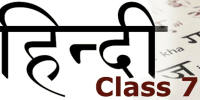## RD Sharma Solutions for Class 7 Mathematics

97 docs

### Top Courses for Class 7Track your progress, build streaks, highlight & save important lessons and more!

,

,

,

,

,

,

,

,

,

,

,

,

,

,

,

,

,

,

,

,

,

,

,

,

,

,

,

,

,

,

;